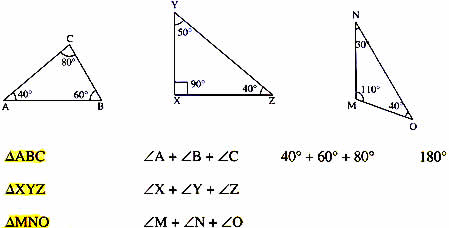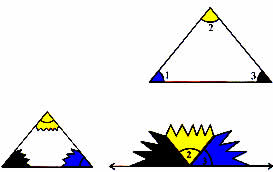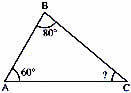Thursday , July 7 2022# NCERT 5th Class (CBSE) Mathematics: Polygons And Circles

## TRIANGLES

### Properties of Triangles

A. Find the sum of the angles in these triangles.In all three triangles, the sum of the measures of the angles is 180°. This is a property of all triangles.

The sum of the measures of the three angles of a triangles is always 180°.

You can check this out for yourself.

1. Draw and cut out a triangle.
2. Colour the three angles of the triangle.
3. Tear off the three corners of the triangle.
4. Place them together like this.

All three pieces fit together to from a straight angle.

∠1 + ∠2 + ∠3 = 180°Look at triangles ABC. The measures of ∠A and ∠B are given. What is the measure of ∠C ?In ΔABC, ∠A + ∠B = 60° + 80° = 140°

The sum of three angles of a triangle is 180°

So, ∠C = 180° – 140° = 40°